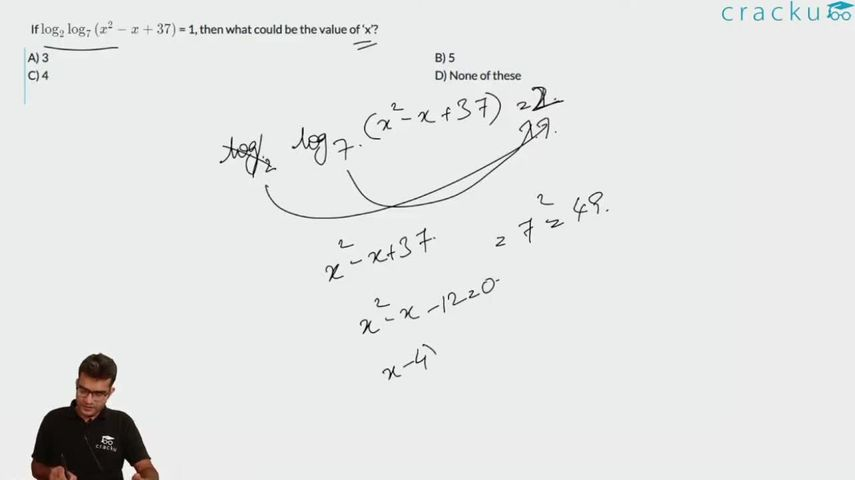Question 39

# If $$\log_{2}{\log_{7}{(x^2 - x+37)}}$$ = 1, then what could be the value of ‘x’?

Solution

$$\log_{2}{\log_{7}{(x^2 - x+37)}}$$ = 1

$$\log_{7}{(x^2 - x+37)}$$ = $$2$$

$$(x^2 - x+37)$$ = $$7^{2}$$

Given eq. can be reduced to $$x^2 - x + 37 = 49$$

So x can be either -3 or 4.

### View Video Solution